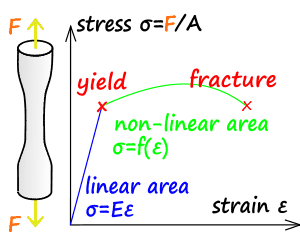## Strength Of Material (SOM) Notes Free Pdf Download

Strength Of material Notes by Prof. M. S. Sivakumar,  Indian Institute of Technology Madras.
This notes are best for strength of material subject.Som Basic
Contents:
1. Introduction
2. Free body diagram – Revisited
3. Normal, shear and bearing stress
4. Stress on inclined planes under axial loading
5. Strain
6. Mechanical properties of materials
7. True stress and true strain
8. Poissons ratio
9. Elasticity and Plasticity
10. Creep and fatigue
11. Deformation in axially loaded members
12. Statically indeterminate problems
13. Thermal effect
14. Design considerations
15. Strain energy
1. Stress at a point
2. Stress Tensor
3. Equations of Equilibrium
4. Different states of stress
5. Transformation of plane stress
6. Principal stresses and maximum shear stress
7. Mohr’s circle for plane stress
Torsion Notes:
1. Introduction
2. Basic Assumptions
3. Torsion
4. Formula
5. Stresses on Inclined Planes
6. Angle of twist
7. Angle of Twist in Torsion
Maximum Stress
8. Torsion of Circular Elastic Bars
• Failure of torsional bars.
• Design of torsional members
• Stress concentration
• Energy
• Inelastic torsional bars
• Non-circular cross section
• Thin walled members
• Numerical solutions
Problem Related To Bending
• Problem 1: Computation of Reactions
• Problem 2: Computation of Reactions
• Problem 3: Computation of Reactions
• Problem 4: Computation of forces and moments
• Problem 5: Bending Moment and Shear force
• Problem 6: Bending Moment Diagram
• Problem 7: Bending Moment and Shear force
•  Problem 8: Bending Moment and Shear force
• Problem 9: Bending Moment and Shear force
• Problem 10: Bending Moment and Shear force
• Problem 11: Beams of Composite Cross Section
Deflection of Beams
• Introduction
• Deflection of Beams (Solution Method by Direct Integration)
• Moment – Area Method for finding Beam Deflections
• Problem 1: Derivation of Shear stress in rectangular crosssection
• Problem 2: Computation of Shear stresses
• Problem 3: Computation of Shear stresses
• Problem 4: Computation of Shear stresses